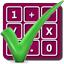#### Arithmetic of Computers

#### from Tenscope Limited

By using this site, you are accepting "session" cookies, as set out in the site's Privacy Policy
Cookies are also used to remember which page of the book you last viewed, so that when you revisit the site you automatically return to the last page you visited.

### Lesson 1

#### Page 1

Electronic digital computers perform complicated logical and arithmetical operations in thousandths or even millionths of a second. Inside the computer, numbers are represented by sequences of electrical pulses. But the number-system used usually differs considerably from that commonly employed in pen-and-paper arithmetic.
To understand how a computer uses its peculiar number system, we shall have to spend some time dissecting and examining more closely the number system we already know.
Our familiar number system uses ten different numerals: 0, 1, 2, 3, 4, 5, 6, 7, 8 and 9. Each single numeral is called a digit. Because the system uses ten different numerals or digits it is called the decimal system (Latin decem = ten). The arithmetic we learned in school is decimal arithmetic.
We are so familiar with the decimal system and decimal arithmetic that the decimal system may seem to us the “natural” system. Actually it is only one of many systems of writing numbers.
Now here is a question on the material you have just read. Select what you believe to be the correct answer and turn to the page number indicated to the right of the answer you choose.
Would you say that the two numbers 492 and .29 are both written in the decimal system?